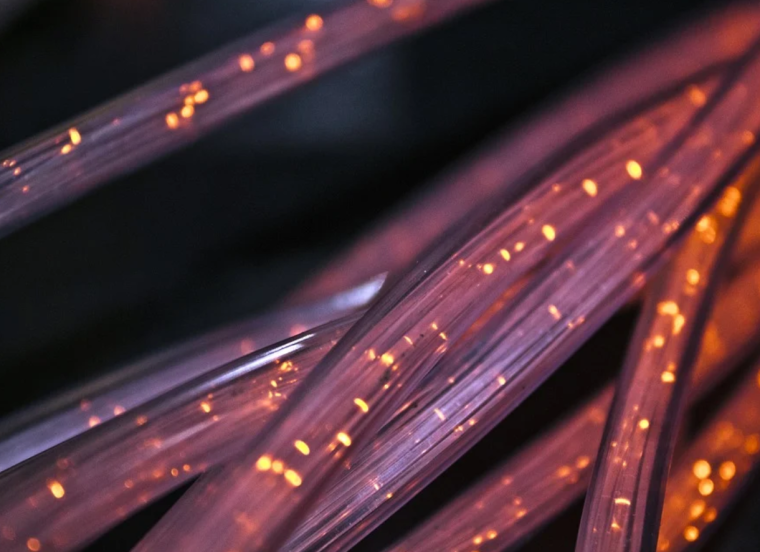# 电线电缆成本计算公式，算出合理的工程造价

10-14 1阅读先看各个电线电缆线材成本核算标准

单价(元/Km)=人工成本+原料成本+水电成本+设备折旧成本+包装成本

一、 人工成本(C1)：人工成本(元/Km)=(D×K÷V÷T÷60÷F÷S)×(1+A)×1000

D：操作员的日薪(元/人日)K：成品中该制程的条数，以LAN Cable为例，芯线制程为8，对绞为4，集合与外被为1; V：制程中机器的线速(M/min);T：一天的工时，以12小时计(hr/日);F：制程中机器的操作率(%)S：每人操作台数(台/人)A：间接人工成本(%)

二、 原料成本(C2)：原料成本(元/Km)=U×B×(1+E)

U：原料单价(元/Kg)B：原料用量(Kg/ Km)E：制程中原料消耗量(%)

三、 水电成本(C3)：水电成本(元/Km)=P×T×R×G÷V÷T÷60÷F

P：制程中机器的用电量(Kw);T：一天的工时，以12小时计(hr/日);R：用电汇率(元/Kw hr)G：用电比率(%);V：制程中机器的线速(M/min)F：制程中机器的操作率(%)

四、 设备仪器折旧成本(C4)：设备仪器折旧成本(元/Km)H÷(Y×12×25)÷(V×24×60×F)

H：设备仪器取得金额(元)Y：设备仪器折旧年数(年);V：制程中机器的线速(M/min)F：制程中机器的操作率(%);备注：检验仪器之V与F参照外被押出机

五、 包装成本(C5)：包装成本(元/Km)=K÷L×1000

K：包装材料单价(元/个)L：每个包装之线材单长(M/个)

承接电线电缆加工工程一般报价

C=直接材料成本C1+加工成本C2

C1=原材料用量M×原材料单价P1;

C2=机时H1×加工单价P2+人时H2×加工单价P3

H1(h/km)=(1/r/60)×1000×N H1=h10+h11+……+h18+h19

(r为线速m/min ; N为电线次数)

H2(h/km)=机时H/单个人所开机台数量N H2=h21+h22+…..+h29+h20

则依工序不同而有所不同:

束绞人时h21=束绞机时h11/ 7; 绝缘人时h22=绝缘机时h12/ 1;

对绞人时h23=对绞机时h13/ 7; 缭绕人时h24=缭绕机时h14/ 10;

中被人时h25=中被机时h15/ 1; 返捻人时h26=返捻机时h16/ 6;

立式包带人时h27=立式包带机时h17/ 5; 集合人时h28=集合机时h18/ 2;

编织人时h29=编织机时h19/ 6; 外被人时h20=外被机时h10/ 1.

电线电缆材料用量计算公式

1.单导体A单股材料用量(kg/km)==(πd2/4)×σ×C×λ2×λ3 (捻入率(%)=√((πD’/P)2+1) ;d：单股铜线径(mm) ; σ：铜比重=8.9 ;C：电缆芯数;λ2：对绞捻入率(%);λ3：集合捻入率(%);D’：层心径(mm);P：对绞平均绞距或集合绞距(mm)

2.绞铜线:材料用量(kg/km)=(πd2/4)×σ×N×C×λ1×λ2×λ3 (N：铜绞线股数 ;λ1：铜绞线捻入率(%) )

3. 绝缘: 单股材料用量(kg/km)=(π(D2-d2)/4)×σ×C×λ2×λ3

4.绞线 材料用量(kg/km)=((D2-d2)/4+0.3048×d2/4)×π×σ×C×λ2×λ3 (d：绞线径(mm);D：绝缘外径(mm) )

5.包带(绕包) 材料用量(kg/km)=W×T×σ (W：带宽(mm);T：带厚(mm);σ：带模拟重 )

6.包带( 卷包) 材料用量(kg/km)=(D+T)×π×T×σ×(N/(N±1)) (D：集合外径(mm);N：重迭或分开率之倒数，重迭：-，分开：+ )

7.被覆 材料用量(kg/km)=(D2-d2+d2×K)/4×π×σ (d：被覆前芯线外径(mm);D：被覆后完成外径(mm);

K：管型押出K=0，半管型押出K=0.15～0.2，充实押出K=0.2～0.3

8. 编织 材料用量(kg/km)=(πd2/4)×N×C×σ×λ (λ2=π2×(D+5d)2/(25.4×C/2/P)2+1;P=(tan(A)×C×25.4)/(2×π×D) ; A=cos-1((C×N×d)/(2×π×D×F));F=1-√(1-K) ; d：编织单股铜线径(mm) ;N：每锭铜线条数(6～9条);C：锭数(16锭或24锭) ; σ：镀锡铜比重 8.8;P：每吋交叉花数;D：编织前线径(mm);A：编织角度(°);F：单向编织率(%);K：编织遮蔽率(%)

电线电缆制程一般损耗率：

一﹐束绞 0.20%﹔二﹐绝缘0.50%﹔三﹐集合0.20%﹔四﹐立式包带0.10%﹔五﹐编织0.10%﹔六﹐外被2.20%﹔七﹐剪线1.80%

电线电缆公式中常用到的材料比重参数：

一般材料比重﹕Cu-8.89;AL/MY-3.69*10-5;PVC-1.45;充麻-0.47(1.775g/m);棉线-0.75(0.288g/m);PP膜-0.74﹔

以上就是建筑界造价频道为您带来的“电线电缆成本计算公式，算出合理的工程造价”的内容，建筑界造价频道也将持续关注更多工程造价的相关内容，欢迎关注我们，关注建筑界~